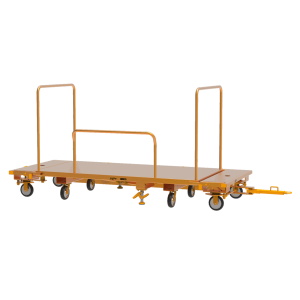# Material Handling Tip: Finding a Load’s Center of Gravity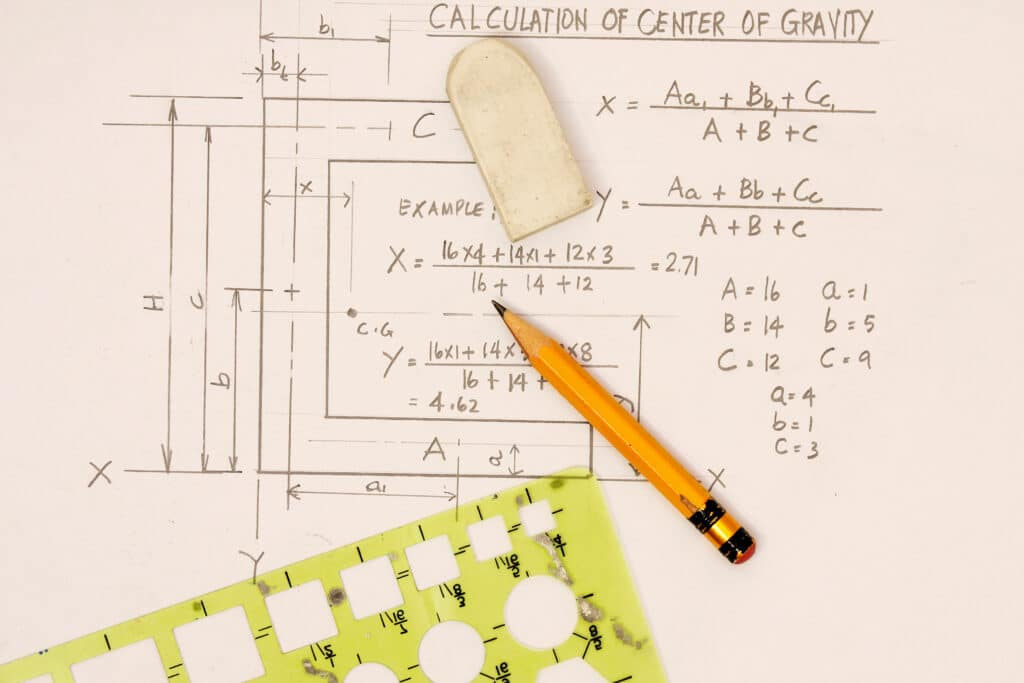# Material Handling Tip: Finding a Load’s Center of Gravity

Learn the importance of finding the center of gravity of a load along with step-by-step instructions on calculating the center of gravity (CoG) or balance point of unusually shaped loads.

In material handling, having a well-balanced pallet can often make the difference between a smooth trip from point A to point B and a costly tip over. Finding this equilibrium is fairly intuitive if you’re moving items that are uniform in shape and weight. However, what do you do when moving objects that don’t have an obvious middle?

## What is the Center of Gravity of a Load?

In physics, an object’s center of gravity, center of mass, or balance point is an imaginary point where there is an equal mass on all sides. In the context of material handling, the Center of Gravity (CoG) refers to the single point of the load or object where it can be carried perfectly balanced. To look at it another way, it’s also the point at which all the load’s weight is concentrated.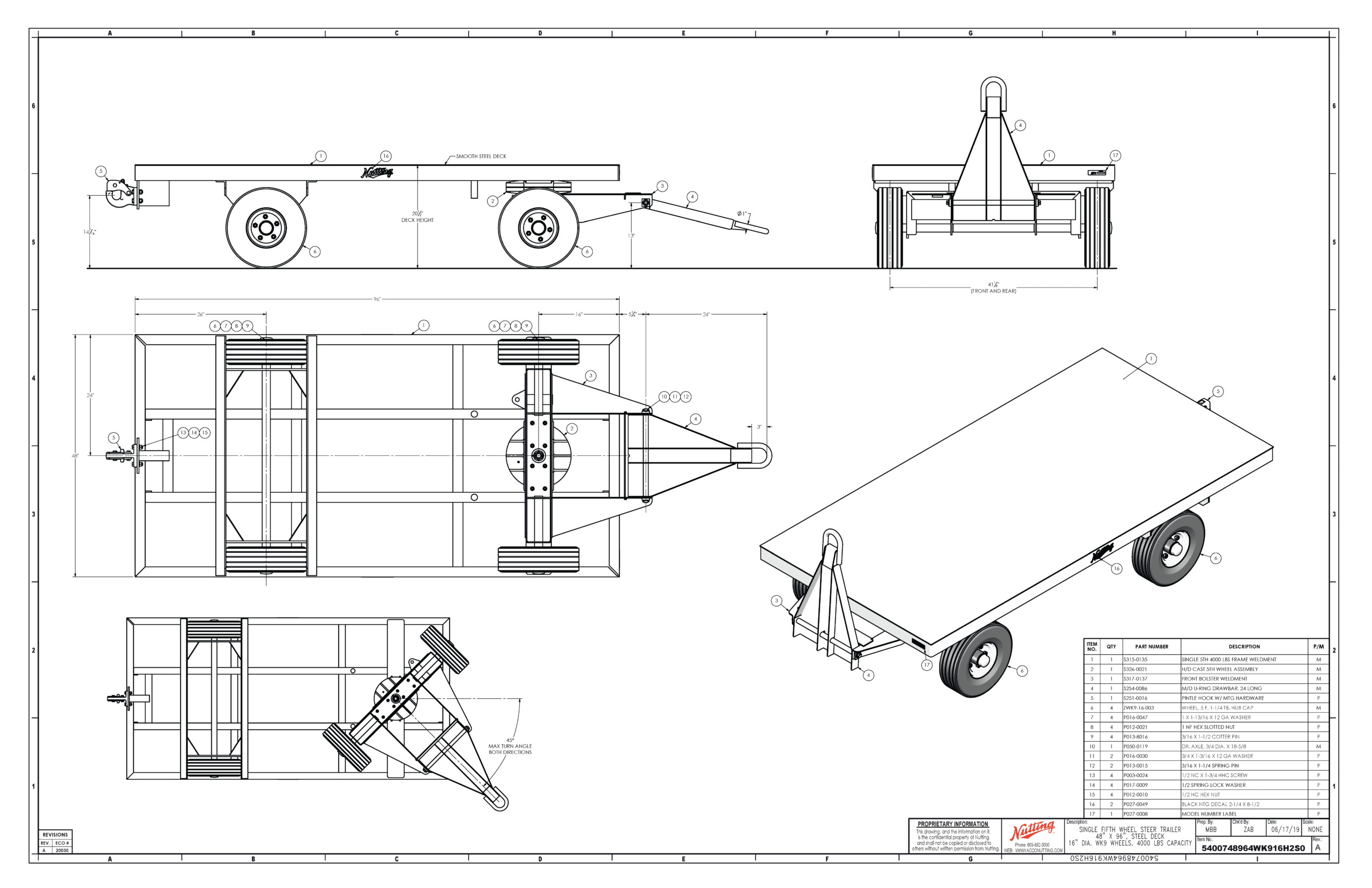## Finding the Location of the Center of Gravity

While there is always the trial and error method, picking different spots and doing test lifts until you find the right location, there are shortcuts you can take to finding the center of gravity on a load without an apparent midpoint. Here are step-by-step instructions on finding the center of gravity of an object or load you need to move around the warehouse using lift scales, a tape measure, and a calculator:

### 1. Determine Load Weight Distribution

Determining weight distribution is where the lift scale comes into play. First, you’ll need to weigh one side of the load, then the other. This will give you the object’s left end weight (LEW), Right End Weight (REW), then combine them for the total weight of the object. The center of gravity shifts towards the heavier side, so knowing these weights is a critical step.

### 2. Divide the Heaviest End by the Total Weight

You only need to calculate one side. Take the heaviest weight between LEW and REW and divide it by the total weight. The ratio of the total weight of an object with its heavy end determines the pivot point of the load. This is where the CoG resides.

### 3. Measure the Span of the Load

The next step is to measure the span of the load. The span is the distance between the two pick points you weighed on either side. Using a tape measure, gauge the distance between pick points and apply the ratio from the previous step to calculate where along that span, the CoG lies.

### 4. Practice Tests

Once you have your CoG measurement, we recommend practice tests where you try lifting the load with a jack or crane a few inches at a time to make sure it’s balanced. If it doesn’t retain its stability and starts to tip or swing, set it back down, adjust the pick point, and try again.

### Example

In this example, we have a load with a LEW of 2,000 lbs, a REW of 1,000, and a total weight of 3,000 lbs. The span is 100 inches. Since the Left End Weight is greater, we divide that by the total weight of 3,000 lbs.

LEW/TW = X

2,000/3,000 = 0.67

Now, take the span of 100 inches and multiply it by the ratio of the weights.

(LEW / TW) x 100” = CoG

(0.67) x 100” = 67”

The CoG is at the 67-inch mark for this load, closer to the heavier side.

In summation, finding the center of gravity for an irregular load can be done through trial and error. Still, some simple math and measurements can significantly reduce the time spent finding that balance.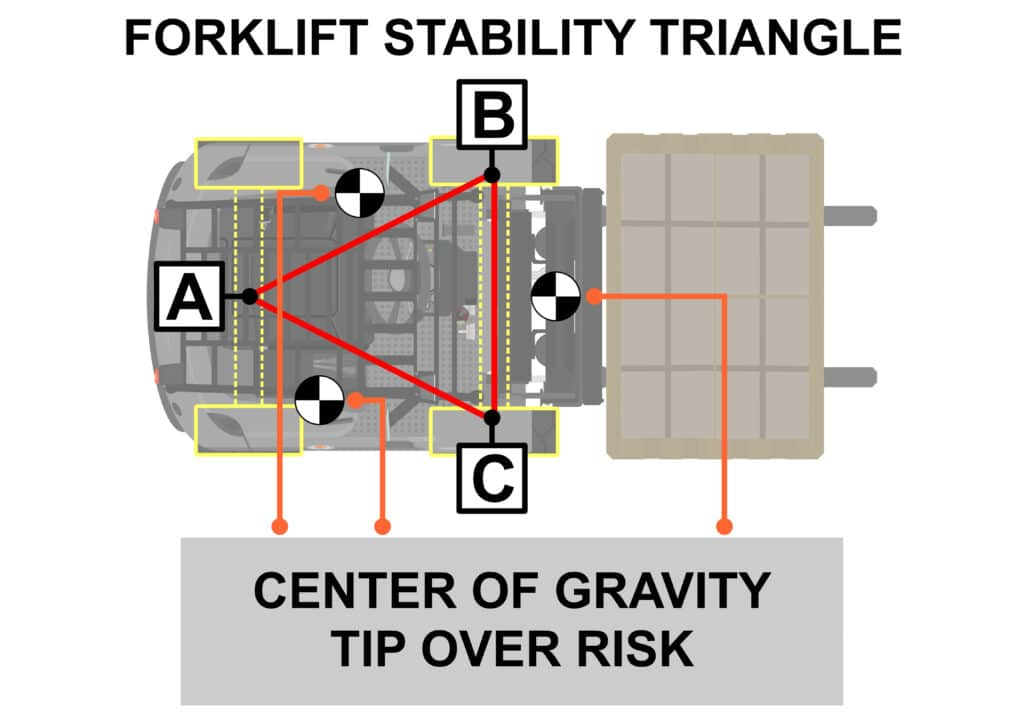At Nutting, we engineer our products to seamlessly integrate with your existing operation with carts that can be customized with various features to accommodate different load types. Does your team produce oddly shapped parts? We can help provide a solution for your needs, all complete with a calculated center of gravity in your production prints.

Knowing which options are best for your application can save you time and money in the long run. That’s why it’s essential to consult an expert before purchasing.

Nutting has over 100 years of experience designing and manufacturing high-quality pallet carts, order picking carts, industrial carts, and more so you can be confident that you’re getting the best possible product for your needs. Contact us today to learn more about our pallet carts and how they can improve operational efficiency in your warehouse or distribution center.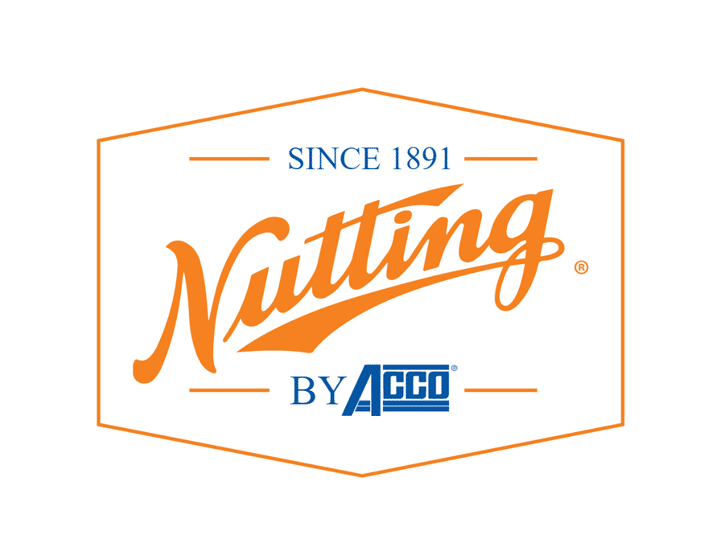### Share Post

•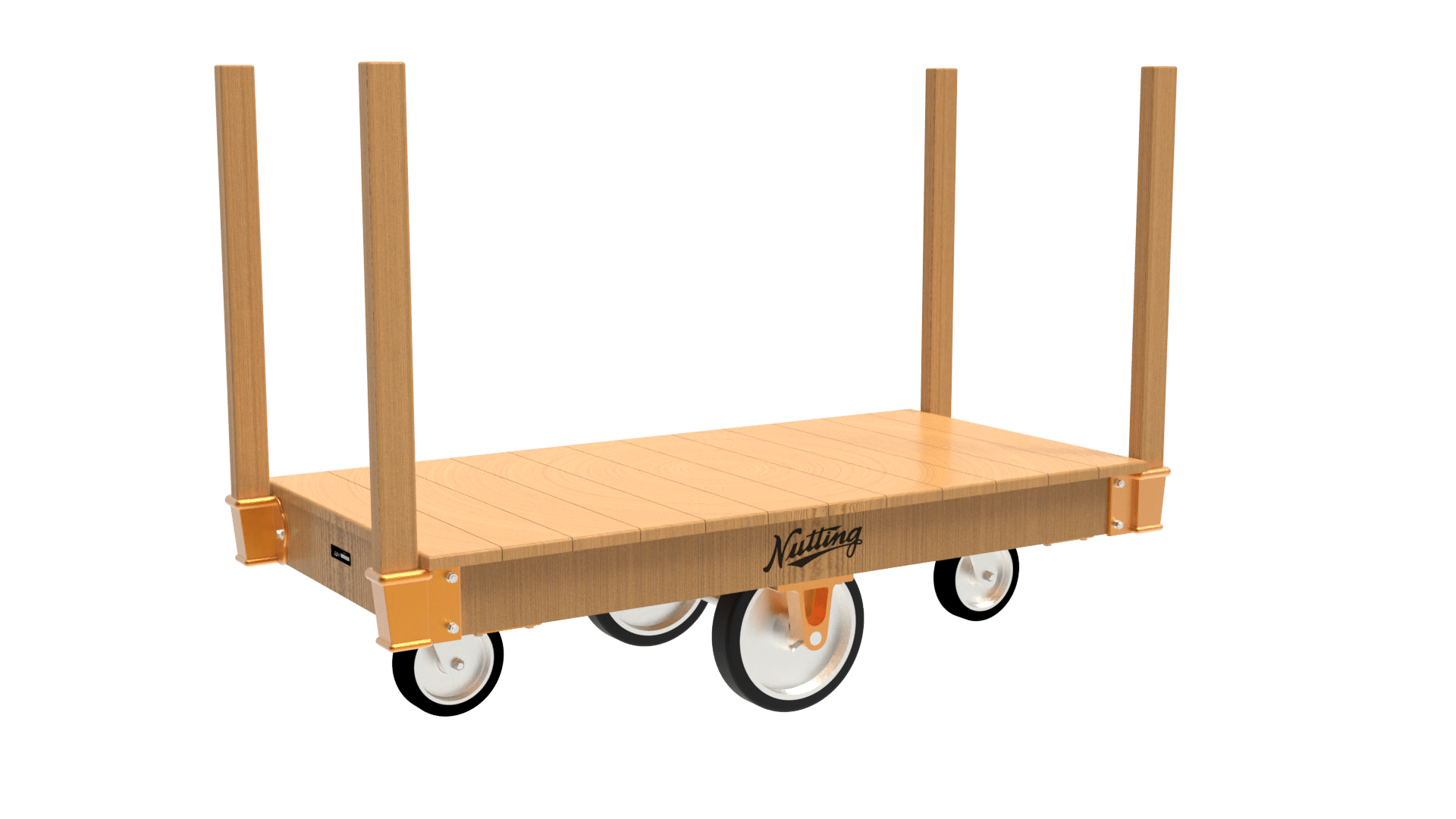Sale!
•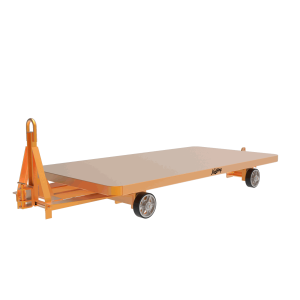•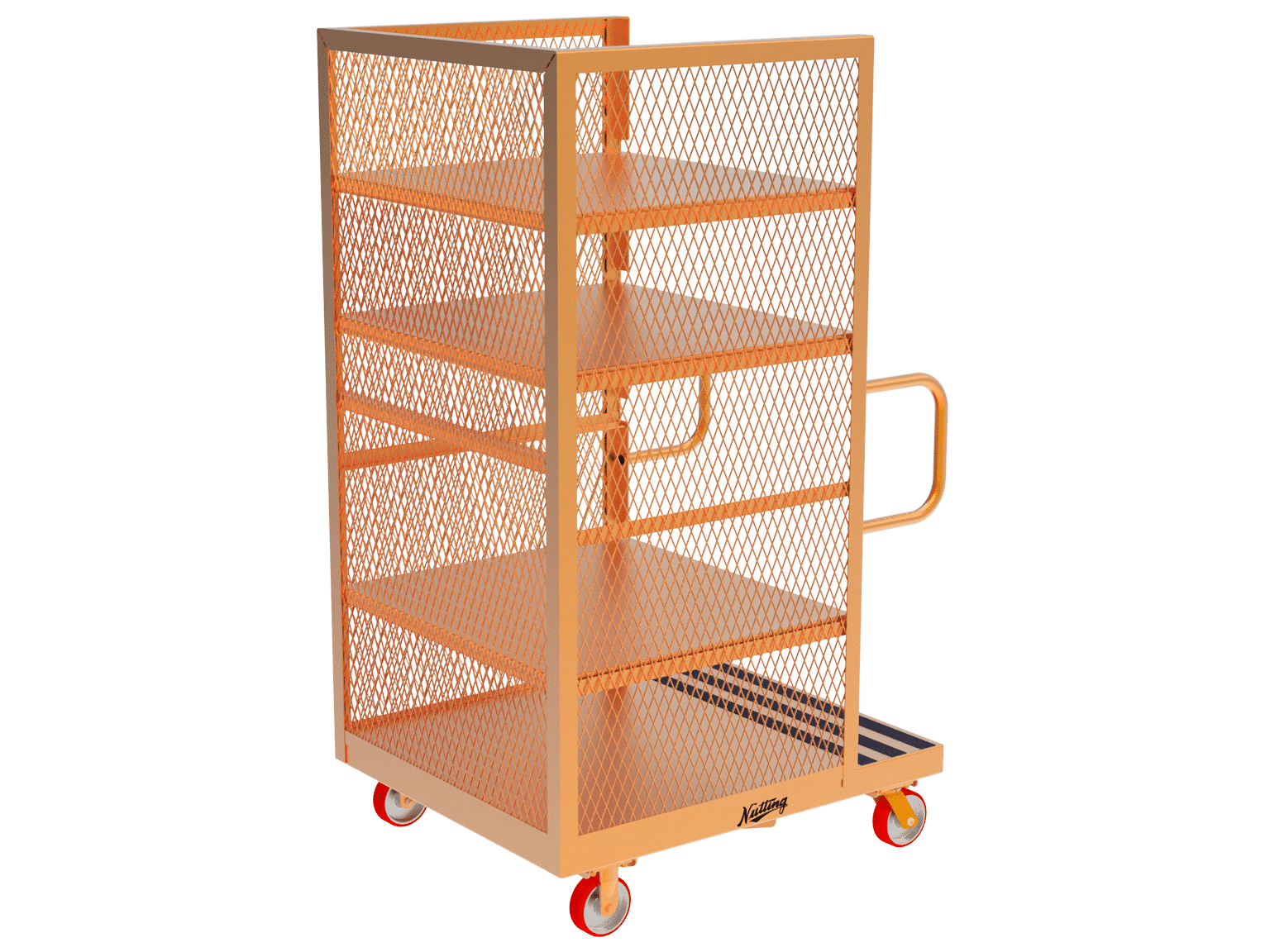•